## RS Aggarwal Class 6 Solutions Chapter 24 Bar Graph Ex 24

These Solutions are part of RS Aggarwal Solutions Class 6. Here we have given RS Aggarwal Solutions Class 6 Chapter 24 Bar Graph Ex 24

Question 1.
Solution:
(i) The given bar graph shows the marks obtained by a student in each of the four subjects in an examination.
(ii) The student is poorest in Science.
(iii) The student is best in Mathematics.
(iv) He got more than 40 marks in Hindi and Mathematics.

Question 2.
Solution:
(i) The given bar graph shows the number of members in each of the 60 families of a colony.
(ii) 10 families have 3 members each.
(iii) 5 couples have no child.
(iv) A family of 4 members is most common.

Question 3.
Solution:
(i) The production was maximum in the 2nd week.
(ii) The production was minimum in the 4th week.
(iii) The average production is 720 per week.
(iv) 2400 cycles were produced in the first three weeks.

Question 4.
Solution:
(i) The given bar graph shows the different modes of transport to a school used by 51 students of a locality.
(ii) Maximum number of students use bicycle for going to school.
(iii) 14 students use bus for going to school.
(iv) 37 students do not use bus for going to school.

Hope given RS Aggarwal Solutions Class 6 Chapter 24 Bar Graph Ex 24 are helpful to complete your math homework.

If you have any doubts, please comment below. Learn Insta try to provide online math tutoring for you.

## RS Aggarwal Class 6 Solutions Chapter 23 Pictograph Ex 23

These Solutions are part of RS Aggarwal Solutions Class 6. Here we have given RS Aggarwal Solutions Class 6 Chapter 23 Pictograph Ex 23

Question 1.
Solution: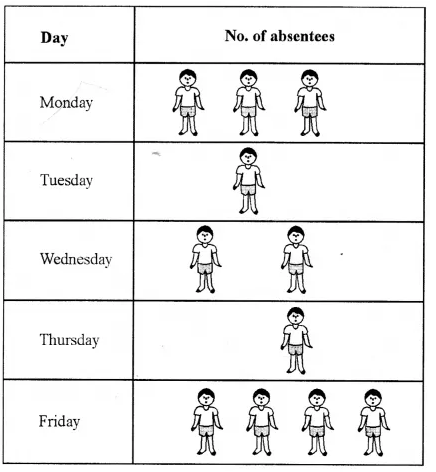Question 2.
Solution:Question 3.
Solution:Question 4.
Solution: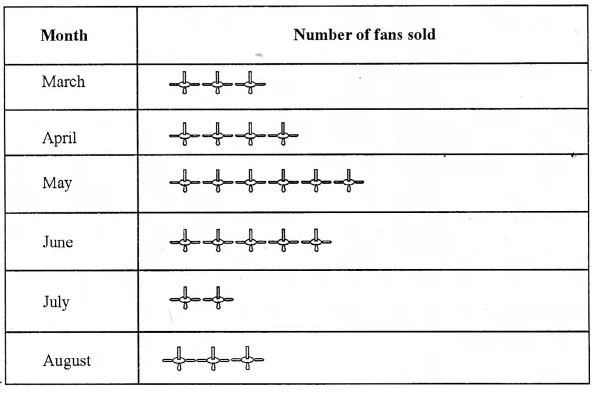Question 5.
Solution:
(i) 24
(ii) 32
(iii) 40
(iv) 96 Ans.

Question 6.
Solution:
(i) 30
(ii) 24
(iii) Friday = 42
(iv) Saturday = 12 Ans.

Hope given RS Aggarwal Solutions Class 6 Chapter 23 Pictograph Ex 23 are helpful to complete your math homework.

If you have any doubts, please comment below. Learn Insta try to provide online math tutoring for you.

## RS Aggarwal Class 6 Solutions Chapter 22 Data Handling Ex 22

These Solutions are part of RS Aggarwal Solutions Class 6. Here we have given RS Aggarwal Solutions Class 6 Chapter 22 Data Handling Ex 22

Question 1.
Solution:
(i) Data. The word data means information in the form of numerical figures.
(ii) Raw data. Data obtained in the original form is called raw data.
(iii) Array. Arranging the numerical figures (data) in an order i.e. ascending or descending order is called an array.
(iv) Tabulation of data. Arranging the given data in a systematic form in the form of a table is called tabulation of the data.
(v) Observations. Each numerical figure in a data is called an observation.
(vi) Frequency of an observation. The number of times a particular observation occurs is called its frequency.
(vii) Statistics. Statistics is a science which deals with the collection, presentation, analysis and interpretation of numerical data.

Question 2.
Solution:
Arranging the given data in ascending order, we get :
0, 0, 1, 1, 1, 1, 1, 1,2, 2, 2, 2,2, 2,2, 2, 2, 3, 3, 3, 3, 3, 4, 4, 4Question 3.
Solution:
Below is given the frequency distribution table of the given data :Question 4.
Solution:
Below is given the frequency distribution table of the given data :Question 5.
Solution:
Below is given the frequency distribution table of the given data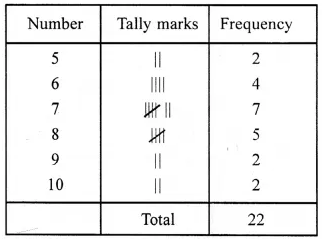Question 6.
Solution:
(i) numerical figures
(ii) original
(iii) array
(iv) frequency
(v) tabulation

Hope given RS Aggarwal Solutions Class 6 Chapter 22 Data Handling Ex 22 are helpful to complete your math homework.

If you have any doubts, please comment below. Learn Insta try to provide online math tutoring for you.

## RS Aggarwal Class 6 Solutions Chapter 21 Concept of Perimeter and Area Ex 21E

These Solutions are part of RS Aggarwal Solutions Class 6. Here we have given RS Aggarwal Solutions Class 6 Chapter 21 Concept of Perimeter and Area Ex 21E

Other Exercises

Objective questions
Mark against the correct answer in each of the following :

Question 1.
Solution:
Ratio in the sides of a rectangle = 7 : 5
and perimeter = 96 cmQuestion 2.
Solution:
Area of a rectangle = 650 cm²
and breadth (b) = 13 cmQuestion 3.
Solution:
Length of a rectangular field (l) = 34 m
and breadth (b) = 18 m
Circumference = 2 (l + b)
= 2 (34 + 18)m
= 2 x 52
= 104 m
Rate of fencing = Rs. 22.50 per m
Total cost = Rs. 22.50 x 104
= Rs. 2340 (b)

Question 4.
Solution:
Total cost of fencing = Rs. 2400
Rate = Rs. 30 per m
Perimeter of the rectangular fieldQuestion 5.
Solution:
Area of rectangular carpet =120 cm²
Perimeter = 46 m
Now 2 (l + b)
= 46 mQuestion 6.
Solution:
Let width of a rectangle = x
Then length = 3x
and diagonal = 6√10 cmQuestion 7.
Solution:
Ratio in length and perimeter of a rectangle = 1 : 3
Let length = x,
then perimeter = 3xQuestion 8.
Solution:
Length of diagonal of a square = 20 cmQuestion 9.
Solution:
Total cost of fencing around a square field = Rs. 2000
and rate = Rs. 25 per metreQuestion 10.
Solution:
Circumference = πd
= $$\\ \frac { 22 }{ 7 }$$ x 7
= 22 cm (b)

Question 11.
Solution:
(a) Diameter = $$\frac { circumference }{ \pi }$$
= $$\\ \frac { 88\times 7 }{ 22 }$$
= 28 cm

Question 12.
Solution:
Circumference = πd
= $$\\ \frac { 22 }{ 7 }$$ x 70
= 220 cmQuestion 13.
Solution:
Length of the lane = 150 m
Breadth of the lane = 9 m
Area of the lane = (150 x 9) m²
= 1350 m²
Area of the brick = 22.5 cm x 7.5 cm
= 168.75 cm²Question 14.
Solution:
Length of a rectangular room (l) = 5 m 40 cm = 5.4 m
and breadth (b) = 4 m 50 cm
= 4.5 m
Area = l x b = 5.4 x 4.5 m²
= 24.3 m² (b)

Question 15.
Solution:
Length of a sheet (l) = 72 cm
and breadth (b) = 48 cm
Area = l x b = 72 x 48 cm²
Area of paper for one envelope = 18 x 12 cm²
No. of envelopes = $$\\ \frac { 72\times 48 }{ 18\times 12 }$$ =16 (d)

Hope given RS Aggarwal Solutions Class 6 Chapter 21 Concept of Perimeter and Area Ex 21E are helpful to complete your math homework.

If you have any doubts, please comment below. Learn Insta try to provide online math tutoring for you.

## RS Aggarwal Class 6 Solutions Chapter 21 Concept of Perimeter and Area Ex 21D

These Solutions are part of RS Aggarwal Solutions Class 6. Here we have given RS Aggarwal Solutions Class 6 Chapter 21 Concept of Perimeter and Area Ex 21D

Other Exercises

Question 1.
Solution:
(i) Length (l) = 46 cm
Area of rectangle = l x b
= 46 x 25 sq. cm
= 1150 sq.cm.
(ii) Length (l) = 9 m
Area = l x b = 9 x 6
= 54 sq. metre Ans.
(iii) Length (l) = 14.5 m
Area = l x b
= 14.5 x 6.8 sq. m
= 98.6 sq. m Ans.
(iv) Length (l) = 2m 5cm
= 2x 100 cm + 5 cm
= 200 cm + 5 cm
= 205 cm
Area = l x b
= 205 cm x 60 cm
= 12300 cm²
(v) Length (l) = 3.5 km
Area = l x b
= 3.5 x 2
= 7 sq. km. Ans.

Question 2.
Solution:
Side of a square plot = 14 m
Area = (Side)²
= (14)²
= 196 m²

Question 3.
Solution:
Length of top of table (l) = 2 m, 25 cm = 2.25 m
Breadth (l) = 1 m 20 cm = 1.20 m
Area of the top of the table = l x b
= (2.25 x 1.20) sq. m
= 2.7 sq. m. Ans.

Question 4.
Solution:
Length of carpet (l) = 30 m 75 cm
= 30.75 m
Breadth (b) = 80 cm = 0.80 m
Area of the carpet = l x b
= (30.75 x 0.80) sq. m
= 24.6 sq. m
Cost of one square metre = Rs. 20
Total cost = 24.6 x 150
= Rs. 3690. Ans

Question 5.
Solution:
Length of the sheet of paper 3 m 24 cm
= 300 cm + 24 cm
= 324 cm
Breadth of the sheet of the paper 1 m 72 cm
= 100 cm + 72 cm
= 172 cm
Area of the sheet of paper = (324 x 172) cm²
Also, area of the piece of paper required for an envelope = (18 x 12) cm².
Number of envelopes that can be made
= $$\\ \frac { 324\times 172 }{ 18\times 12 }$$
= 258

Question 6.
Solution:
Length of room (l) = 12.5 m
Area = l x b
= (12.5 x 8) sq. m
= 100 sq. m
Side of square carpet (a) = 8 m
Area of carpet = a² = (8 x 8) sq. m.
= 64 square metre
Area left without carpet = 100 sq. m – 64 sq. m
= 36 sq. m Ans.

Question 7.
Solution:
Length of the lane = 150 m
Breadth of the lane = 9 m
Area of the lane = (150 x 9) m²
= 1350 m²
Area of the brick = 22.5 cm x 7.5 cm
= 168.75 cm²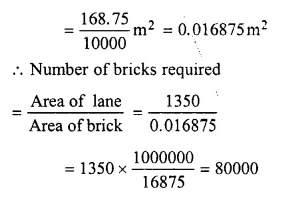Question 8.
Solution:
Length of room (l) = 13 m
Area of floor or carpet = l x b
= 13 x 9
= 117 sq. m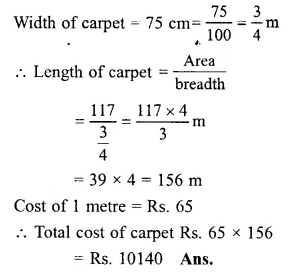Question 9.
Solution:
Let the length of the rectangular park = 5x metres
and the breadth of the rectangular park = 3x metres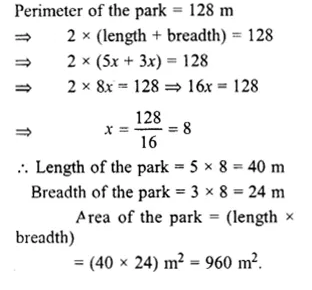Question 10.
Solution:
Side of the square plot = 64 m
Perimeter of the square plot = 4 x Side
= 4 x 64
= 256 m
Perimeter of the rectangular plot = Perimeter of the square plot = 256 m
Length of the rectangular plot = 70 m
Perimeter = 2 x (Length + Breadth)
256 = 2 (70 + b)
256 = 140 + 2b
=> 2b = 256 – 140
=> 2b = 116
b = $$\\ \frac { 116 }{ 2 }$$ = 58 cm
Area of the rectangular plot = (length x breadth)]
= (70 x 58) m²
= 4060 m²
Area of the square plot = Side x Side
= (64 x 64) m²
= 4096 m².
Square plot has the greater area than that of the rectangular plot by
(4096 – 4060)
= 36 m².

Question 11.
Solution:
Total cost of cultivating the rectangular field = Rs. 71400
Rate of cultivating = Rs. 35 per sq. m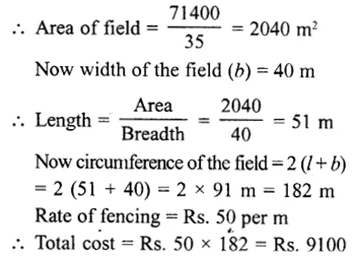Question 12.
Solution:
Area of rectangle = 540 sq. cm
Length (l) = 36 cmQuestion 13.
Solution:
Measure of a marble tile = 12cm x 10cm
Area of wall = 4 m x 3 m
= 12 m²
Area of one marble tile
= 12 x 10
= 120 cm²Question 14.
Solution:
Area of a rectangle = 600 cm²Question 15.
Solution:
diagonal of square = 5 √2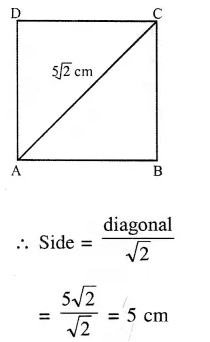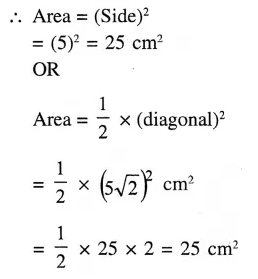Question 16.
Solution:
(i) We name the given region as shown in the figure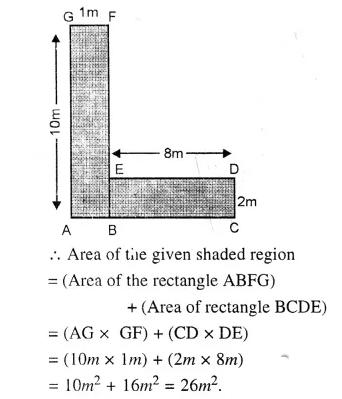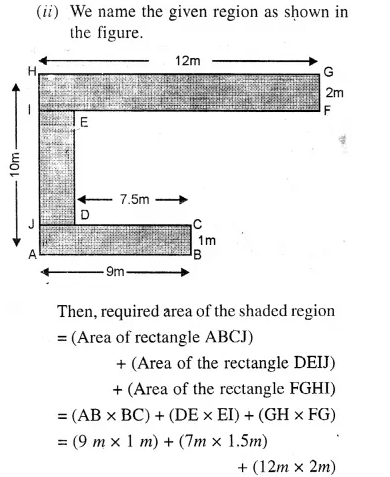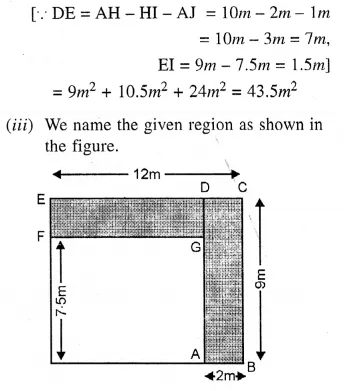Question 17.
Solution:
Measures are in cm
(i) In the figure, there are three rectangles and one square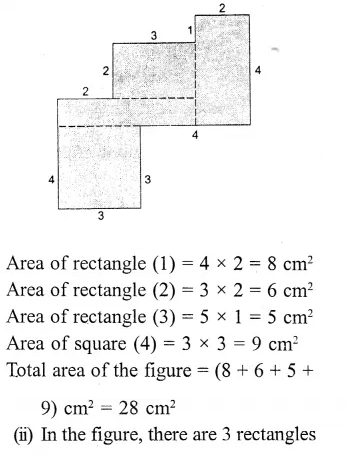= 5(6 x 6) cm²
= 180 cm²

Hope given RS Aggarwal Solutions Class 6 Chapter 21 Concept of Perimeter and Area Ex 21D are helpful to complete your math homework.

If you have any doubts, please comment below. Learn Insta try to provide online math tutoring for you.

## RS Aggarwal Class 6 Solutions Chapter 21 Concept of Perimeter and Area Ex 21C

These Solutions are part of RS Aggarwal Solutions Class 6. Here we have given RS Aggarwal Solutions Class 6 Chapter 21 Concept of Perimeter and Area Ex 21C

Other Exercises

Question 1.
Solution:
1. In the given figure, there are 12 complete squares, so its area is 12 cm².
2. In the given figure, there are 18 complete squares, so its area is 18 cm².
3. In the given figure, there are 14.5 complete squares, so its area is 14.5 cm² .
4. In the given figure, there are 6 complete squares and four half parts of a square, the area of the figure is 8 cm².
5. The given figure contains 9 complete squares and 6 half parts of a square. So the area of the figure is
$$\left( 9+\frac { 6 }{ 2 } \right)$$ = 9 + 3 = 12 cm².
6. The given figure contains 16 complete squares. So, its area is 16 cm².
7. The given figure contains 4 complete squares, 8 more than half parts and 4 less than half parts of a square. Neglecting the less than half parts and considering the more than half parts as complete squares, the approximate area of the figure is 12 cm².
8. The given figure contains 7 complete squares and 5 more than half parts and some less than half parts of a square. Neglecting the less than half parts and considering more than half parts as complete square, the area of the figure is 12 cm² approximately.
9. The given figure contains 14 complete squares and four half parts of a square. So, the area of the figure is
$$\left( 14+\frac { 4 }{ 2 } \right)$$ cm² = (14 + 2) cm² = 16 cm².

Hope given RS Aggarwal Solutions Class 6 Chapter 21 Concept of Perimeter and Area Ex 21C are helpful to complete your math homework.

If you have any doubts, please comment below. Learn Insta try to provide online math tutoring for you.

## RS Aggarwal Class 6 Solutions Chapter 21 Concept of Perimeter and Area Ex 21B

These Solutions are part of RS Aggarwal Solutions Class 6. Here we have given RS Aggarwal Solutions Class 6 Chapter 21 Concept of Perimeter and Area Ex 21B

Other Exercises

Question 1.
Solution:
(i) Radius of the circle (r) = 28 cm
Circumference = 2 πr
= 2 x $$\\ \frac { 22 }{ 7 }$$ x 28 cm
= 176 cm Ans.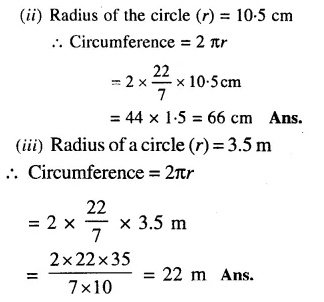Question 2.
Solution:
(i) Diameter of the circle (d) = 14 cm
Circumference = πd
= $$\\ \frac { 22 }{ 7 }$$ x 14
= 44 cm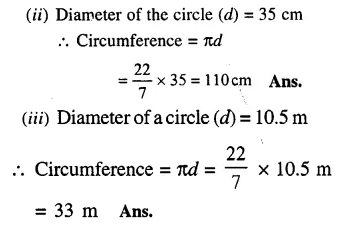Question 3.
Solution:
Circumference of the circle = 176 cm
Let r be the radius, thenQuestion 4.
Solution:
Circumference of a wheel = 264 cm
Let d be its diameter, then
πd = 264
=> $$\\ \frac { 22 }{ 7 } d$$
= 264Question 5.
Solution:
Diameter of the wheel (d) = 77 cm
Circumference = πdQuestion 6.
Solution:
Diameter of the wheel = 70 cm
circumference = πdHope given RS Aggarwal Solutions Class 6 Chapter 21 Concept of Perimeter and Area Ex 21B are helpful to complete your math homework.

If you have any doubts, please comment below. Learn Insta try to provide online math tutoring for you.

## RS Aggarwal Class 6 Solutions Chapter 5 Fractions Ex 5C

These Solutions are part of RS Aggarwal Solutions Class 6. Here we have given RS Aggarwal Solutions Class 6 Chapter 5 Fractions Ex 5C.

Other Exercises

Question 1.
Solution:
(i) $$\\ \frac { 2 }{ 3 }$$
= $$\\ \frac { 2X2 }{ 3X2 }$$
= $$\\ \frac { 4 }{ 6 }$$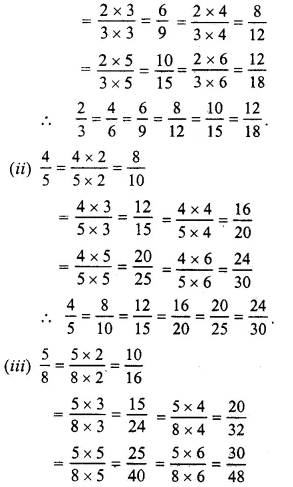Question 2.
Solution:
(i) In $$\\ \frac { 5 }{ 6 }$$ and $$\\ \frac { 20 }{ 24 }$$
$$\\ \frac { 5 }{ 6 }$$ = $$\\ \frac { 20 }{ 24 }$$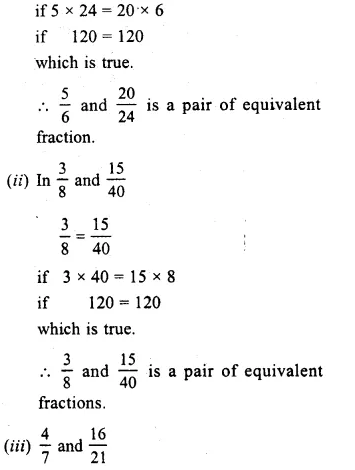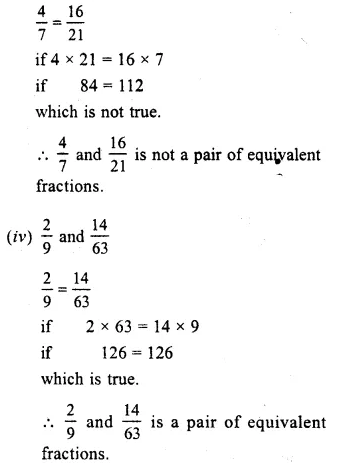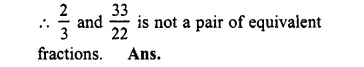Question 3.
Solution:
Equivalent fraction of $$\\ \frac { 3 }{ 5 }$$ having
(i) Denominator = 30 and 30 = 5 x 6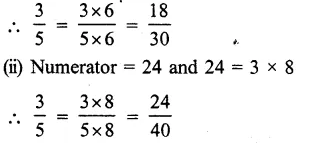Question 4.
Solution:
(i) Denominator = 54, and 54 = 9 x 6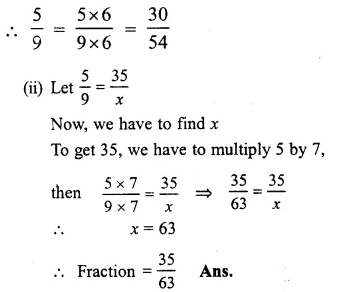Question 5.
Solution:
Equivalent fraction of $$\\ \frac { 6 }{ 11 }$$ having
(i) Denominator = 77 and 77 = 11 = 7
$$\\ \frac { 6 }{ 11 }$$
= $$\\ \frac { 6X7 }{ 11X7 }$$
= $$\\ \frac { 42 }{ 77 }$$
(ii) Numerator = 60 and 60 = 6 x 10
$$\\ \frac { 6 }{ 11 }$$
= $$\\ \frac { 6X10 }{ 11X10 }$$
= $$\\ \frac { 60 }{ 110 }$$

Question 6.
Solution:
Let $$\\ \frac { 24 }{ 30 }$$ = $$\\ \frac { 4 }{ x }$$
In order to get 4, divide 24 by 6,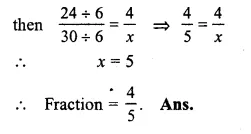Question 7.
Solution:
Equivalent fraction of $$\\ \frac { 36 }{ 48 }$$, with
(i) Numerator 9 and 9 = 36 + 4
$$\frac { 36 }{ 48 } =\frac { 36\div 4 }{ 48\div 4 } =\frac { 9 }{ 12 }$$
(ii) Denominator = 4 and 4 = 48 ÷ 12
$$\frac { 36 }{ 48 } =\frac { 36\div 12 }{ 48\div 12 } =\frac { 3 }{ 4 }$$

Question 8.
Solution:
Equivalent fraction of $$\\ \frac { 56 }{ 70 }$$ with
(i) Numerator 4 and = 56 ÷ 14
$$\frac { 56 }{ 70 } =\frac { 56\div 14 }{ 70\div 14 } =\frac { 4 }{ 5 }$$
(ii) Denominator =10 and 10 = 70 ÷ 7
$$\frac { 56 }{ 70 } =\frac { 56\div 7 }{ 70\div 7 } =\frac { 8 }{ 10 }$$

Question 9.
Solution:
(i) In $$\\ \frac { 9 }{ 15 }$$, HCF of 9 and 15 = 3
Now, dividing each term by 3, we get:
$$\frac { 9 }{ 15 } =\frac { 9\div 3 }{ 15\div 3 } =\frac { 3 }{ 5 }$$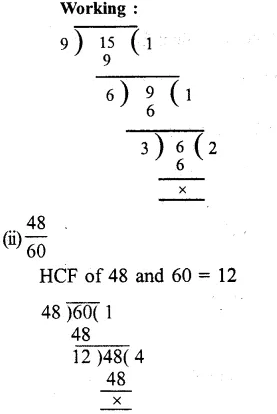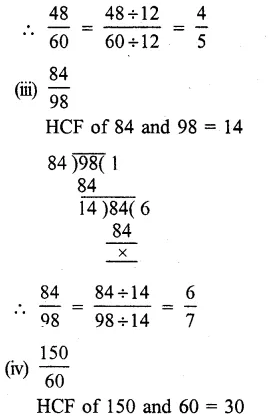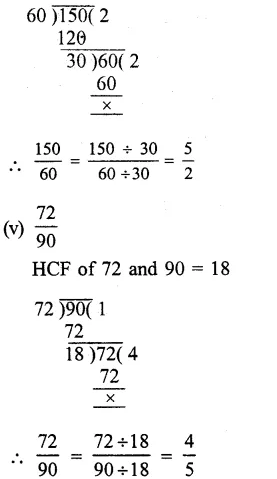Question 10.
Solution:
We know that a fraction is in its simplest form if its HCF of numerator and denominator is 1.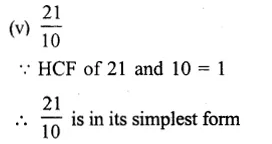Question 11.
Solution: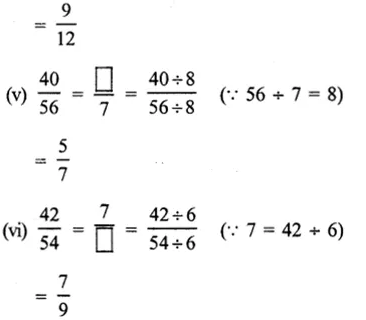Hope given RS Aggarwal Solutions Class 6 Chapter 5 Fractions Ex 5C are helpful to complete your math homework.

If you have any doubts, please comment below. Learn Insta try to provide online math tutoring for you.

## RS Aggarwal Class 6 Solutions Chapter 5 Fractions Ex 5B

These Solutions are part of RS Aggarwal Solutions Class 6. Here we have given RS Aggarwal Solutions Class 6 Chapter 5 Fractions Ex 5B.

Other Exercises

Question 1.
Solution:
We know that, a fraction is proper if its denominator is greater than its numerator. Therefore,
$$\\ \frac { 1 }{ 2 }$$, $$\\ \frac { 3 }{ 5 }$$ and $$\\ \frac { 10 }{ 11 }$$ are proper fractions. Ans.

Question 2.
Solution:
We know that a fraction is improper if its denominator is less than its numerator
Therefore,are improper fractions. Ans.

Question 3.
Solution:
Six improper fractions with denominator 5 can beQuestion 4.
Solution:
Six improper fraction with denominator 13 can be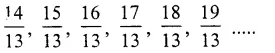Question 5.
Solution: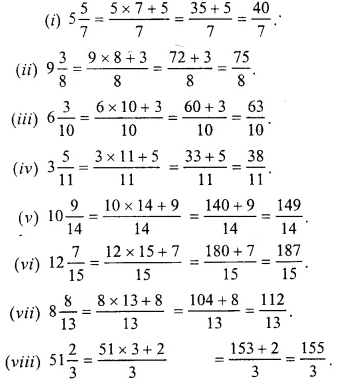Question 6.
Solution: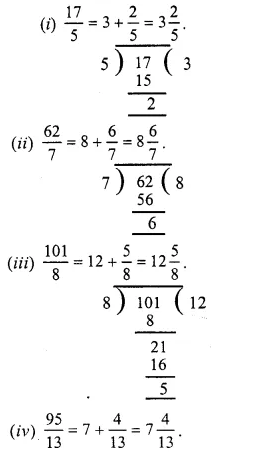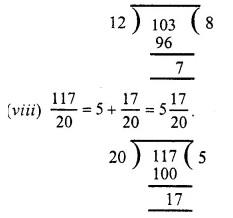Question 7.
Solution: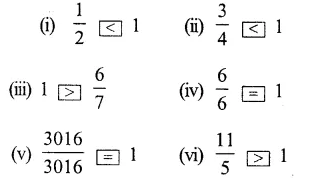Question 8.
Solution:Hope given RS Aggarwal Solutions Class 6 Chapter 5 Fractions Ex 5B are helpful to complete your math homework.

If you have any doubts, please comment below. Learn Insta try to provide online math tutoring for you.

## RS Aggarwal Class 6 Solutions Chapter 5 Fractions Ex 5A

These Solutions are part of RS Aggarwal Solutions Class 6. Here we have given RS Aggarwal Solutions Class 6 Chapter 5 Fractions Ex 5A.

Other Exercises

Question 1.
Solution:
(i) $$\\ \frac { 3 }{ 4 }$$
(ii) $$\\ \frac { 1 }{ 4 }$$
(iii) $$\\ \frac { 2 }{ 3 }$$
(iv) $$\\ \frac { 3 }{ 10 }$$
(v) $$\\ \frac { 4 }{ 9 }$$
(vi) $$\\ \frac { 3 }{ 8 }$$

Question 2.
Solution:
In the figure, $$\\ \frac { 4 }{ 9 }$$ is shaded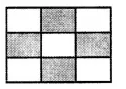Question 3.
Solution:
In the figure, whole rectangle is not divided into four equal parts.

Question 4.
Solution:
(i) Three-fourths = $$\\ \frac { 3 }{ 4 }$$
(ii) Four-sevenths = $$\\ \frac { 4 }{ 7 }$$
(iii) Two-fifths = $$\\ \frac { 2 }{ 5 }$$
(iv) Three-tenths = $$\\ \frac { 3 }{ 10 }$$
(v) One-eighth = $$\\ \frac { 1 }{ 8 }$$
(vi) three-tenths = $$\\ \frac { 5 }{ 6 }$$
(vii) five-sixths = $$\\ \frac { 8 }{ 9 }$$
(vii) seven-twelfths = $$\\ \frac { 7 }{ 12 }$$

Question 5.
Solution:
(i) In $$\\ \frac { 4 }{ 9 }$$, numerator is 4 and denominator is 9.
(ii) In $$\\ \frac { 6 }{ 11 }$$, numerator is 6 and denominator is 11.
(iii) In $$\\ \frac { 8 }{ 15 }$$, numerator is 8 and denominator is 15.
(iv) In $$\\ \frac { 12 }{ 17 }$$, numerator is 12 and denominator is 17.
(v) $$\\ \frac { 5 }{ 1 }$$ , numerator is 5 and denominator is 1.

Question 6.
Solution:
(z) Numerator = 3, Denominator = 8, then fraction = $$\\ \frac { 3 }{ 8 }$$.
(ii) Numerator = 5, Denominator = 12, then fraction = $$\\ \frac { 5 }{ 12 }$$
(iii) Numerator = 7, Denominator = 16, then fraction = $$\\ \frac { 7 }{ 16 }$$.
(iv) Numerator = 8, Denominator = 15, then fraction = $$\\ \frac { 8 }{ 15 }$$

Question 7.
Solution:
(i) $$\\ \frac { 2 }{ 3 }$$ = two-thirds
(ii) $$\\ \frac { 4 }{ 9 }$$ = four-ninths
(iii) $$\\ \frac { 2 }{ 5 }$$ = two-fifths
(iv) $$\\ \frac { 7 }{ 10 }$$ = seven-tenths
(v) $$\\ \frac { 1 }{ 3 }$$ = one-thirds
(vi) $$\\ \frac { 3 }{ 4 }$$ = three-fourth
(vii) $$\\ \frac { 3 }{ 8 }$$ = three-eighths
(viii) $$\\ \frac { 9 }{ 14 }$$ = nine-fourteenths
(ix) $$\\ \frac { 5 }{ 11 }$$ = five-elevanths
(x) $$\\ \frac { 6 }{ 15 }$$ = six-fifteenths

Question 8.
Solution:
24 minutes is the fraction of 1 hour i.e.,
60 minutes = $$\\ \frac { 24 }{ 60 }$$

Question 9.
Solution:
Natural number between 2 to 10 are 2, 3, 4, 5, 6, 7, 8, 9, 10 = 9
Out of these prime number are 2, 3, 5, 7 = 4
Fraction = $$\\ \frac { 4 }{ 9 }$$

Question 10.
Solution:
(i) $$\\ \frac { 2 }{ 3 }$$ of 15 pens = $$\\ \frac { 2 }{ 3 }$$ x 15 = 2 x 5 = 10 pens.
(ii) $$\\ \frac { 2 }{ 3 }$$ of 27 balls = $$\\ \frac { 2 }{ 3 }$$ x 27 = 2 x 9 = 18 balls.
(iii) $$\\ \frac { 2 }{ 3 }$$ of 36 balloons = $$\\ \frac { 2 }{ 3 }$$ x 36 = 2 x 12 = 24 balloons. Ans.

Question 11.
Solution:
(i) $$\\ \frac { 3 }{ 4 }$$ of 16 cups = $$\\ \frac { 3 }{ 4 }$$ x 16 = 3 x 4
= 12 cups.
(ii) $$\\ \frac { 3 }{ 4 }$$ of 28 rackets = $$\\ \frac { 3 }{ 4 }$$ x 28 = 3 x 7
= 21 rackets.
(iii) $$\\ \frac { 3 }{ 4 }$$ of 32 books = $$\\ \frac { 3 }{ 4 }$$ x 32 = 3 x 8
= 24 books. Ans.

Question 12.
Solution:
Total number of pencils Neelam has = 25
No. of pencils given to Meena
= $$\\ \frac { 4 }{ 5 }$$ of 25
= $$\\ \frac { 4 }{ 5 }$$ x 25 – 20
No. of pencils left with Neelam = 25 – 20 = 5

Question 13.
Solution:
(i) $$\\ \frac { 3 }{ 8 }$$
Take a line segment OA = one unit of length
Divide it into 8 equal parts and take 3 parts at P, then P represents $$\\ \frac { 3 }{ 8 }$$.(ii) $$\\ \frac { 5 }{ 9 }$$
(a) Take a line segment OA = one unit of length.
(b) Divide it into nine equal parts and take 5 parts at P, then P represents $$\\ \frac { 5 }{ 9 }$$.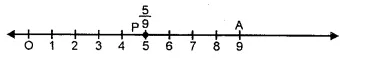(iii) $$\\ \frac { 4 }{ 7 }$$
(a) Take a line segment OA = one unit of length.
(b) Divide it into 7 equal parts and take 4 parts at P then P represents $$\\ \frac { 4 }{ 7 }$$.(iv) $$\\ \frac { 2 }{ 5 }$$
(a) Take a line segment OA = 1 unit of length.
(b) Divide it with 5 equal parts and take 2 parts and P then P represents $$\\ \frac { 2 }{ 5 }$$.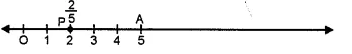(v) $$\\ \frac { 1 }{ 4 }$$
(a) Take a line segment OA = 1 unit of length.
(b) Divide it with 4 equal parts and take 1 parts and P then P represents $$\\ \frac { 1 }{ 4 }$$.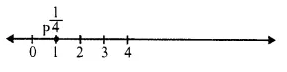Hope given RS Aggarwal Solutions Class 6 Chapter 5 Fractions Ex 5A are helpful to complete your math homework.

If you have any doubts, please comment below. Learn Insta try to provide online math tutoring for you.

## RS Aggarwal Class 6 Solutions Chapter 4 Integers Ex 4F

These Solutions are part of RS Aggarwal Solutions Class 6. Here we have given RS Aggarwal Solutions Class 6 Chapter 4 Integers Ex 4F.

Other Exercises

OBJECTIVE QUESTIONS
Tick the correct answer in each of the following :

Question 1.
Solution:
(b) Because – 4 < – 3.

Question 2.
Solution:
Because – 3 – 2 = – 5.

Question 3.
Solution:
(c) Because 4 + ( – 5) = – 1.

Question 4.
Solution:
(a) Because – 7 – 2 = – 9.

Question 5.
Solution:
(b) Because 7 + | – 3| = 7 + 3 = 10.

Question 6.
Solution:
(c) Because – 42 + ( – 35) = – 42 – 35 = – 77.

Question 7.
Solution:
(b) Because ( – 37) + 6 = – 31.

Question 8.
Solution:
(c) Because 49 + ( – 27) = 49 – 27 = 22.

Question 9.
Solution:
(c) Because successor of – 18 = – 18 + 1 = – 17.

Question 10.
Solution:
(b) Because predecessor of – 16 is = – 16 – 1 = – 17.

Question 11.
Solution:
(a) Because additive inverse of – 5 is = – ( – 5) = 5.

Question 12.
Solution:
(b) Because – 12 – ( – 5) = – 12 + 5 = – 7

Question 13.
Solution:
(b) Because 5 – ( – 8) = 5 + 8 = 13.

Question 14.
Solution:
(c) Because other – 25 – 30 = – 55.

Question 15.
Solution:
(a) Because other 20 – ( – 5) = 20 + 5 = 25.

Question 16.
Solution:
(b) Because other – 13 – 8 = – 21.

Question 17.
Solution:
(b) Because 0 – ( – 8) = 0 + 8 = 8

Question 18.
Solution:
(c) Because 8 + ( – 8) = 8 – 8 = 0.

Question 19.
Solution:
(c)Because- 6 + 4 – ( – 3) = – 6 + 4 + 3 = 7 – 6 = 1.

Question 20.
Solution:
(c) Because 6 – ( – 4) = 6 + 4 = 10.

Question 21.
Solution:
(a) Because ( – 7) + ( – 9) + 12 + ( – 16) = – 7 – 9 + 12 – 16 = – 32 + 12 = – 20.

Question 22.
Solution:
(c) Because – 4 – (8) = – 4 – 8 = – 12.

Question 23.
Solution:
(c) Because – 6 – ( – 9) = – 6 + 9 = 3.

Question 24.
Solution:
(c) Because 10 – ( – 5) = 10 + 5 = 15.

Question 25.
Solution:
(b) Because ( – 6) x 9 = 54.

Question 26.
Solution:
(a) Because ( – 9) x 6 + ( – 9) x 4
= – 54 – 36 = – 90.

Question 27.
Solution:
(b) Because 36 + ( – 9) = $$\\ \frac { 36 }{ -9 }$$ = – 4.

Hope given RS Aggarwal Solutions Class 6 Chapter 4 Integers Ex 4F are helpful to complete your math homework.

If you have any doubts, please comment below. Learn Insta try to provide online math tutoring for you.